【阅读时间】15-20min 7930 words
【阅读内容】使用联想链条和几何直观，辅以从实际需求衍生概念的思考模式，详解什么是傅立叶变换为什么要做傅立叶变换等，帮助记忆和理解，目的当然是标题所说：让你永远忘不了傅里叶变换这个公式。另，这篇博客还从侧面一定程度上回答了另一个问题：为什么要研究复数

# 欧拉公式与旋转

$e^{\pi i}$ 表示的是在单位圆逆时针在旋转180°这个变换

$$e^{\pi i} + 1 = 0$$

## 对称性 symmetry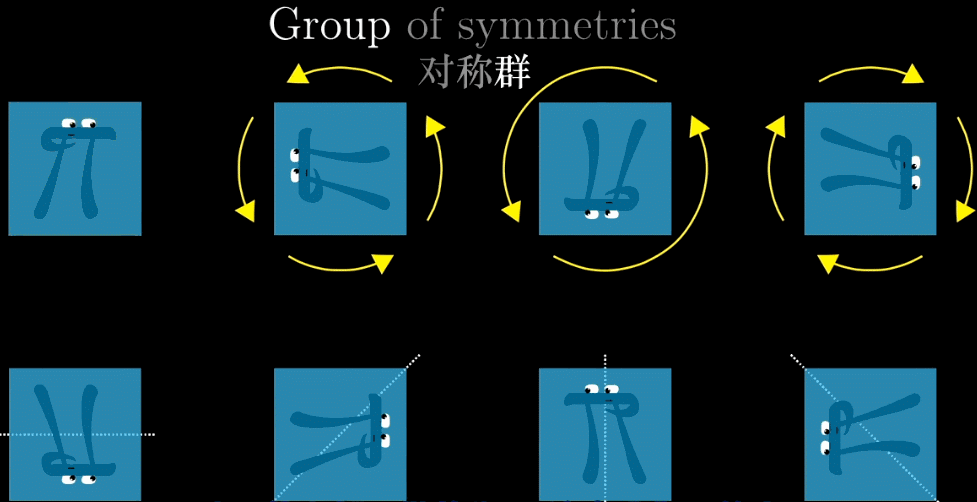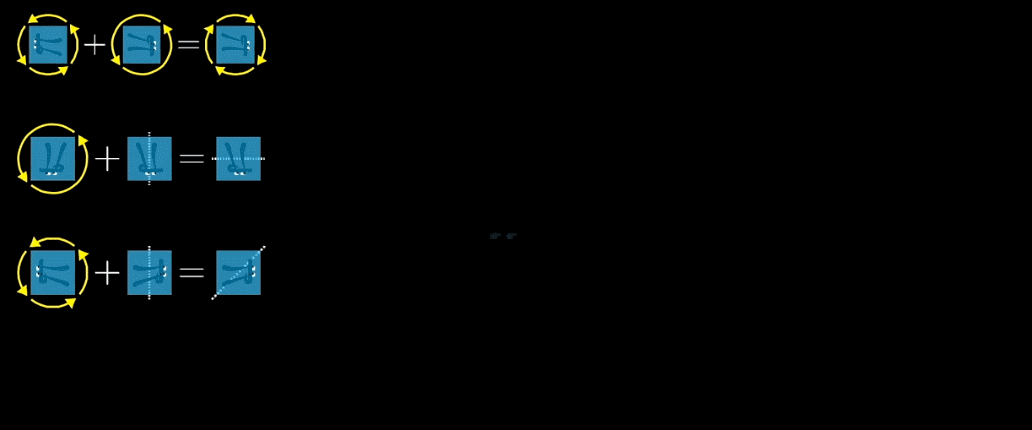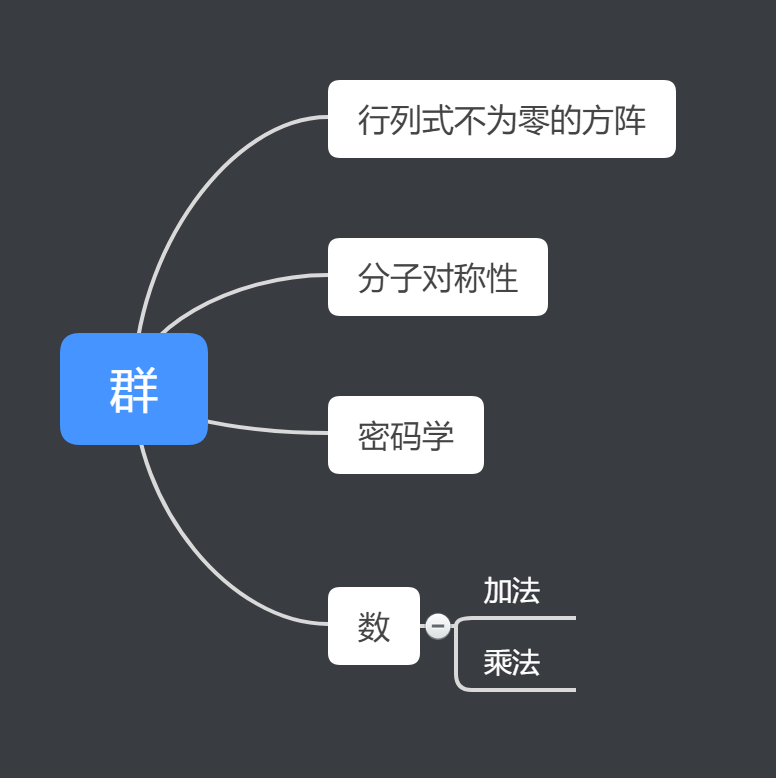1. 想做到把一个点变到另一个点：即从（1,0）通过伸缩旋转到（-1,0），长度不变，只旋转就能实现

2. 此时，注意到了一个形式很有特点的定义： $-1 = i \times i$ ，-1 就是我们需要的目标位置，那如何从（1,0）出发进行两次同样的操作可以得到（-1,0）呢？（这个操作即 $i$ 这个虚数单位定义的操作）答案即：一个单位 $i$ 表示旋转90°即可

3. 更意外的发现，进行一次 $i$ 操作，如果是逆时针旋转90°，正好会落在二维平面y轴的（0,1）与单位长度不谋而合

4. 更大胆的假设，如果y轴自带虚数单位，如 $i,2i,3i……$ ，就有旋转操作了，是不是就就能通过乘法来描述处在这个平面上的所有变换了？

$i$ 怎么可能表示旋转呢？怎么看都不像啊，陷入僵局。那么不妨换个角度来思考，旋转到底是什么？

$$e^x = 1 + x + \frac{1}{2!} x^2 + \frac{1}{3!} x^3 + \cdots \tag{1} \\ sin(x) = x - \frac{1}{3!}x^3 + \frac{1}{5!}x^5 + \cdots \\ cos(x) = 1 - \frac{1}{2!}x^2 + \frac{1}{4!}x^4 + \cdots$$

\begin{align}e^{i\theta} & = 1 + {i\theta} + \frac{1}{2!}({i\theta})^2 + \frac{1}{3!} ({i\theta})^3 + \frac{1}{4!} ({i\theta})^4 + \frac{1}{5!} ({i\theta})^5 + \cdots \\ &= (1 - \frac{\theta^2}{2!} + \frac{\theta^4}{4!} + \cdots) + i(\theta -\frac{\theta^3}{3!} + \frac{\theta^5}{5!} + \cdots) \\&= cos(\theta) + isin(\theta)\end{align}\tag{4}$e^{i\theta}$ 这个公式等价于一种旋转，$\theta$ 为旋转角的度数（统一单位，弧度制，即把°转换成实数）$\theta = 2\pi$ 即为360°，是单位圆

## 指数函数 Exponentiation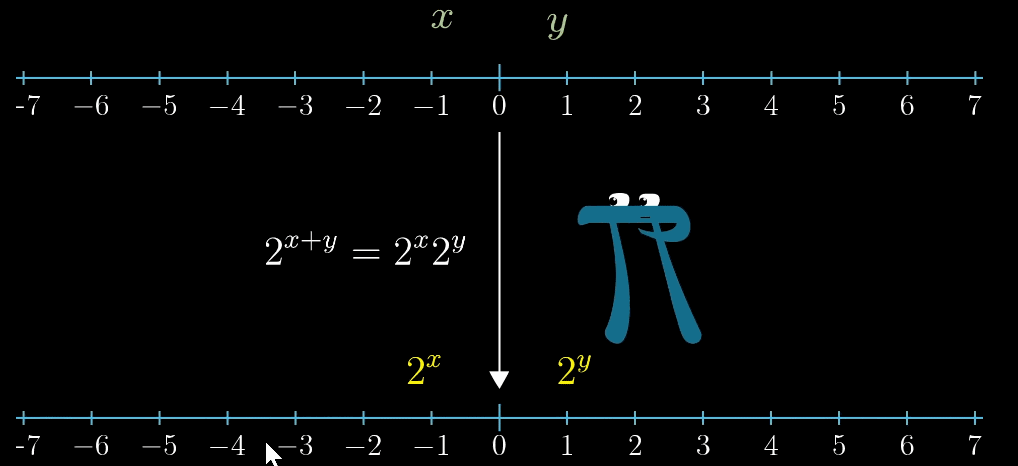## 复平面 Complex Plane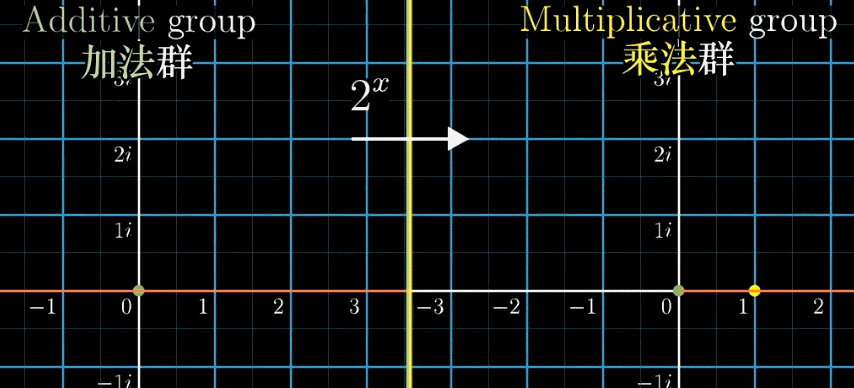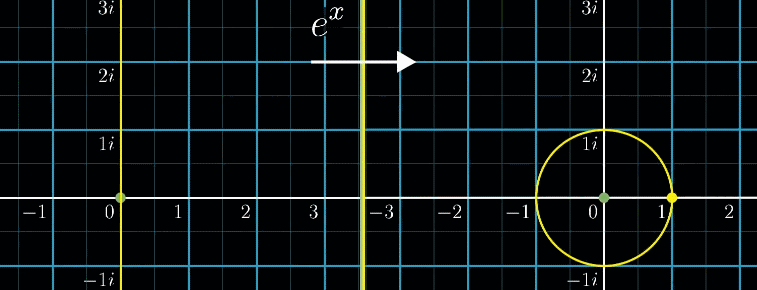## 总结

$e^{x}$ 在复平面，或者说 $x = ai$ （a为某个常数，就是弧度制的圆周长度）代表的变换是：旋转

# 傅立叶变换

## 什么是傅立叶变换

### 公式表示

$$\hat f (\xi) = \int_{-\infty}^{\infty} f(x) e^{-2\pi i x \xi} dx \quad \xi \;为任意实数$$

## 【看到】傅立叶变换

### 声音的表示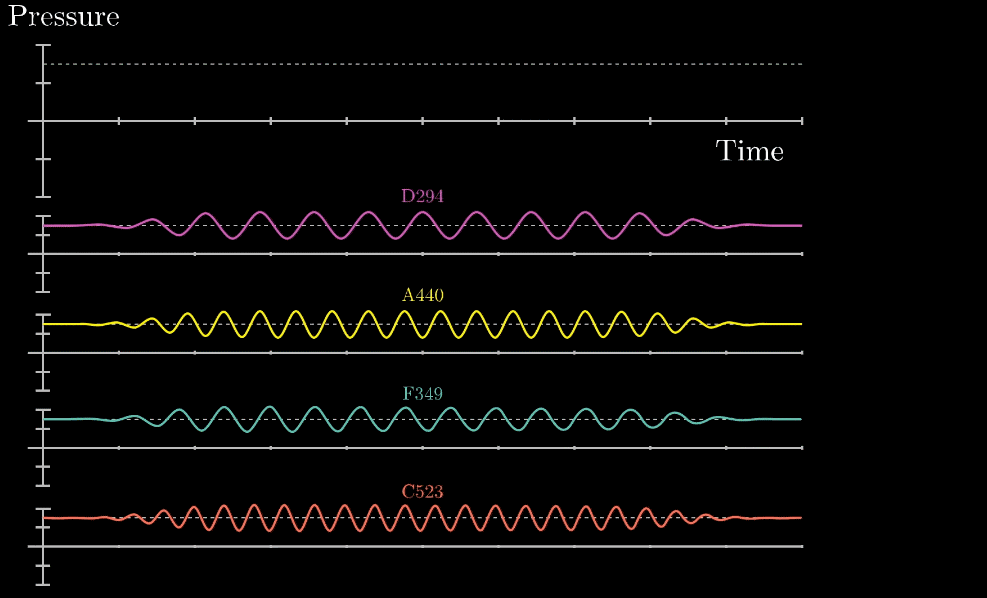### 可视化方法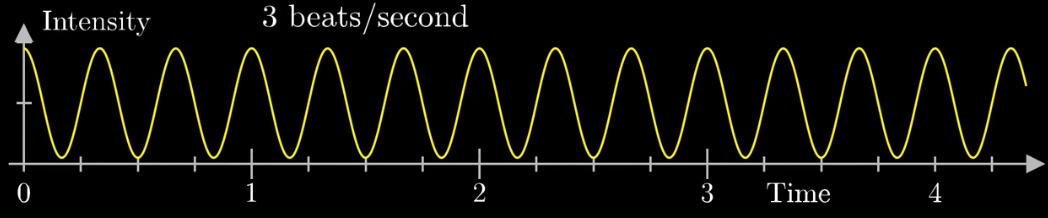#### 绕圈记录法：同一事物的不同角度• 首先把黄色曲线缠绕到一个圆上，大小就是原本信号的振幅
• 圆周围的图像由白色的箭头绘制而成，速度可变，上图中的白色箭头移动速度是每秒钟转过半圈（这个速度是对于下面的圆形图像来说，每秒钟在圆形图像中转半圈），对应上面的则是虚线表示一圈走到的位置，0.5拍子/秒
• 此时，有两个频率在起作用，一个是信号的频率：3次震荡/秒，另一个是图像缠绕中心圆的频率，为0.5圈/秒，第二个频率可以自由改变，相当于一个变量，下面的动图直观的展现了缠绕速度变化时的可视化表现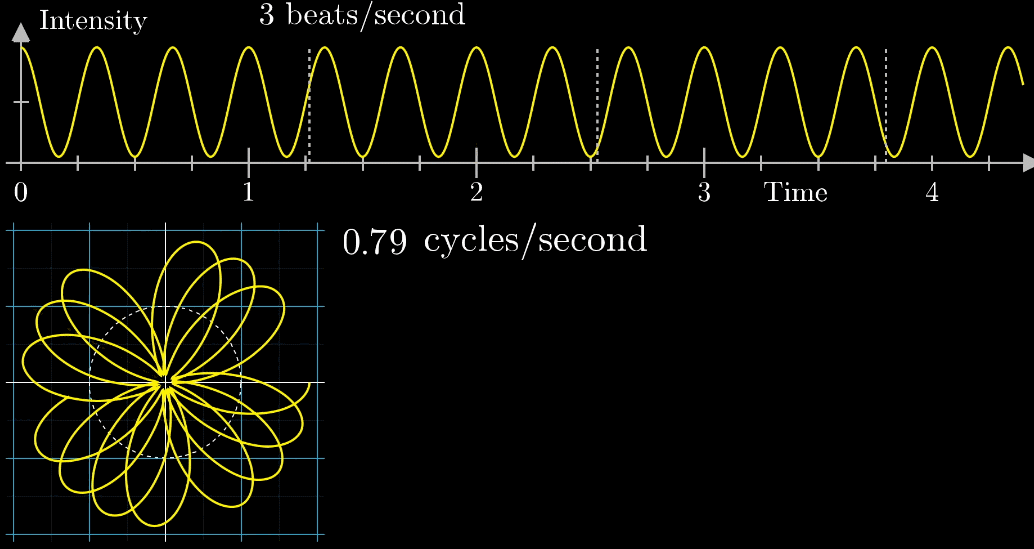#### 质心记录法：新维度的特征提取

（1）自变量是什么？（输入特征）

（2）输出（新的圆圈图）有什么特征？（输出特征）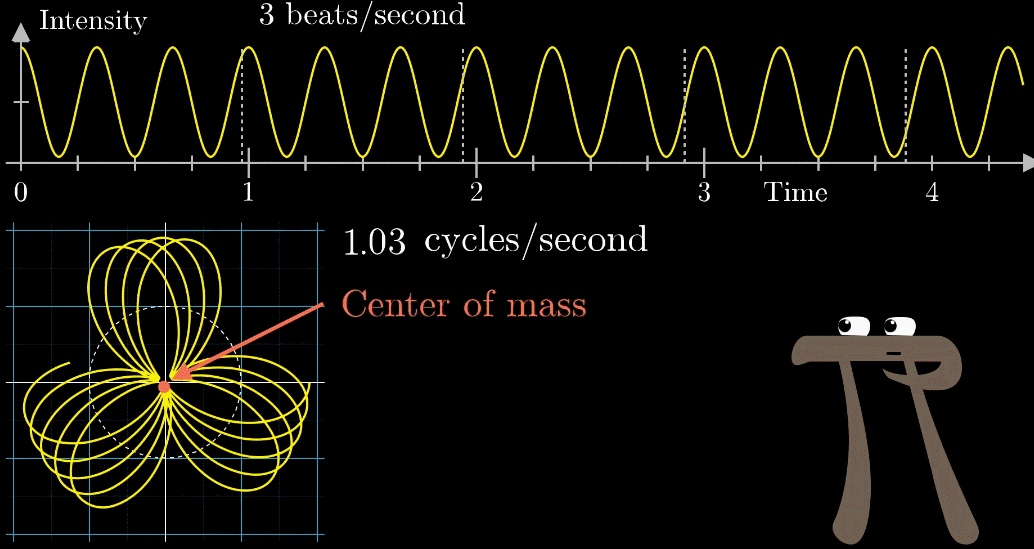【输入（横坐标）】➜【进行采样的（白色箭头）的绕圈速度】

【输出（纵坐标）】➜【圆圈图的质心位置的横坐标】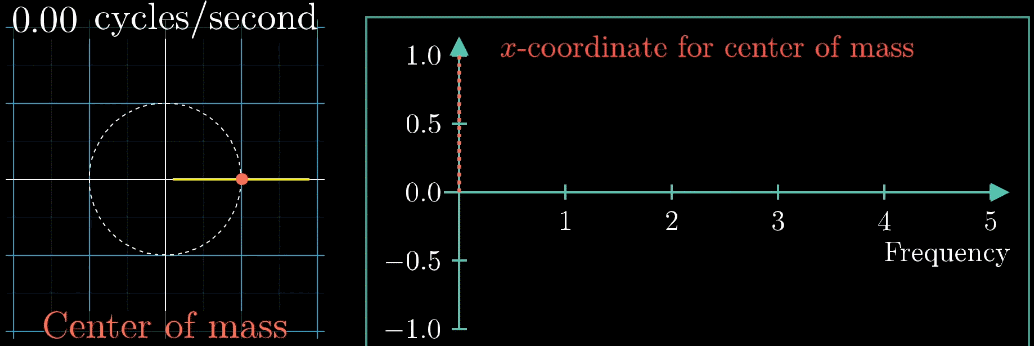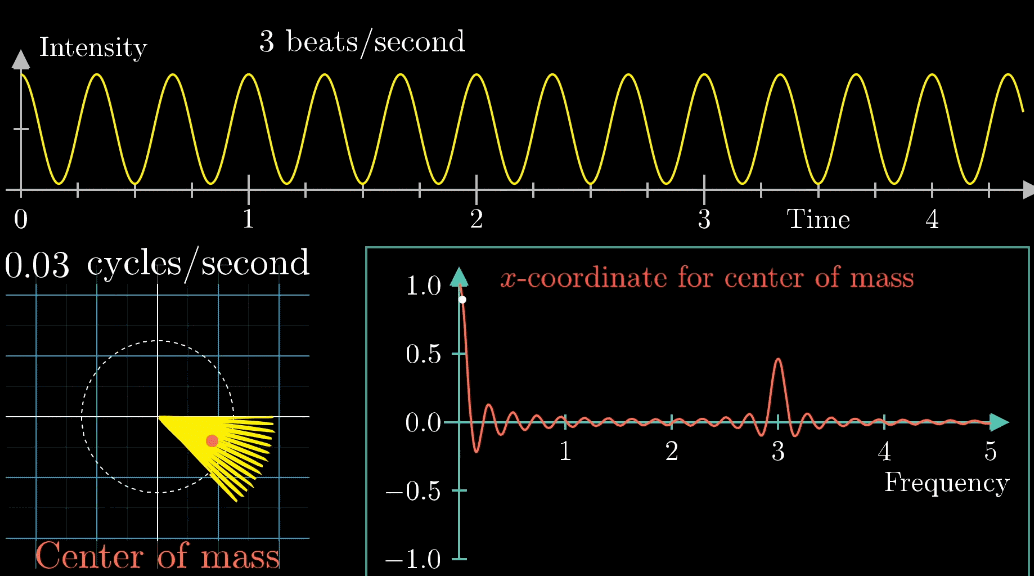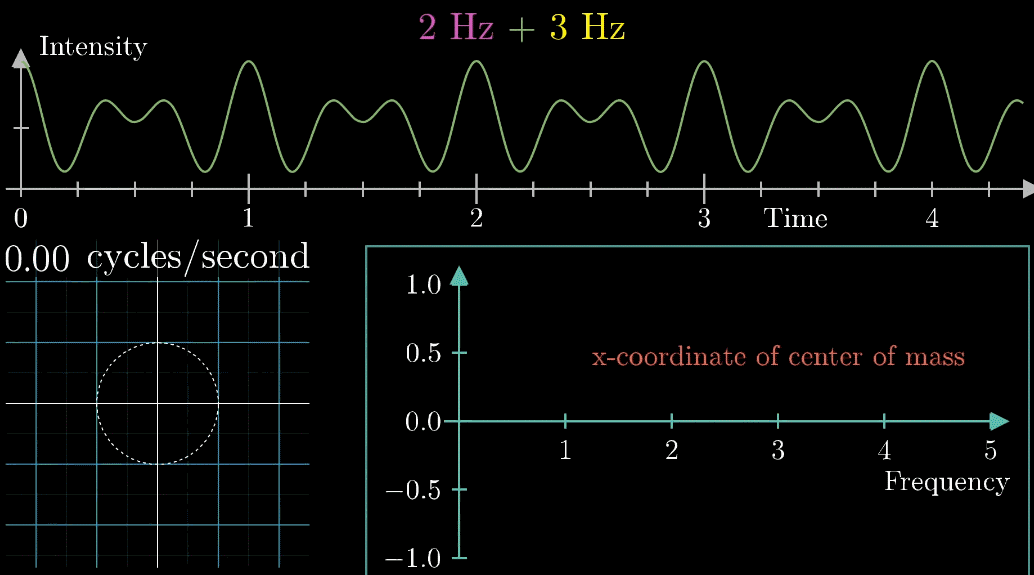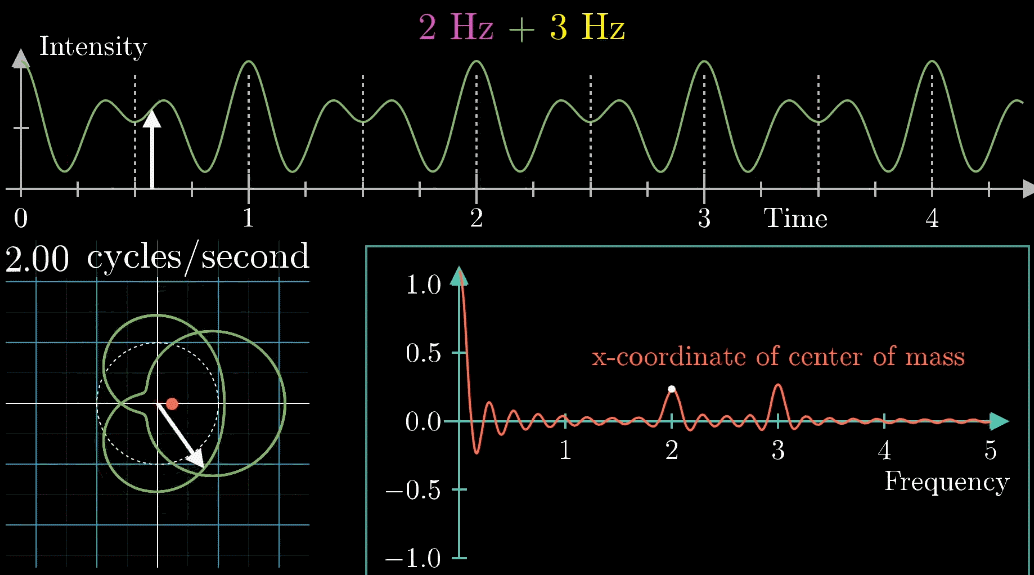BTW，图形的一部分有点像动画EVA中某个使徒的脸，带给人一种诡异的仪式感。数学之令人敬畏，可能在这一刻熠熠生辉，刺的人睁不开眼

### 公式表示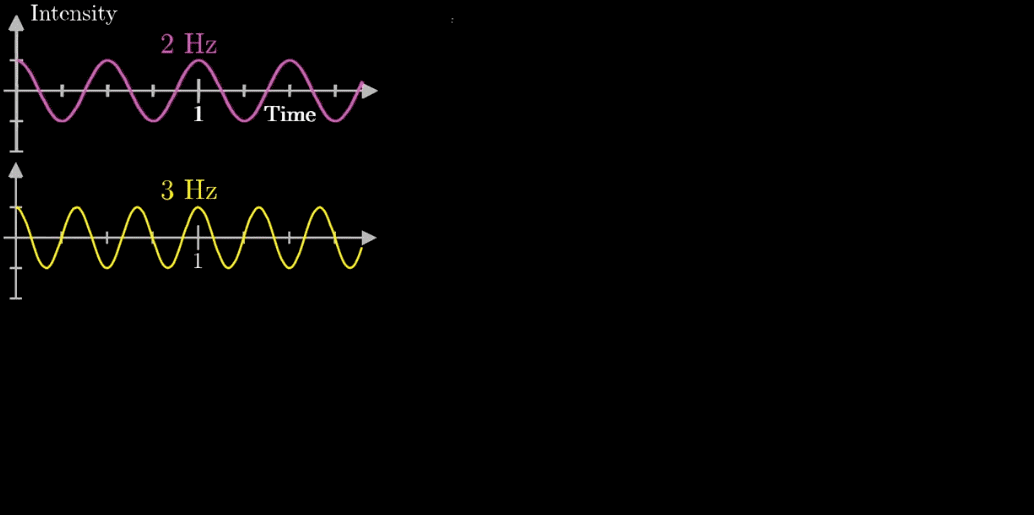#### 第一步：旋转的表示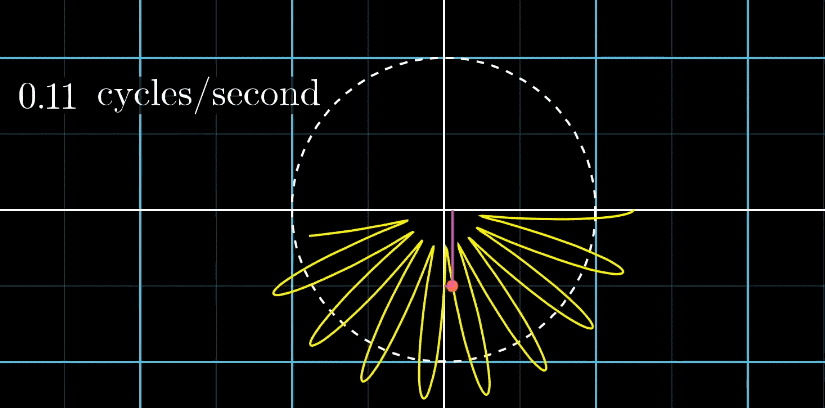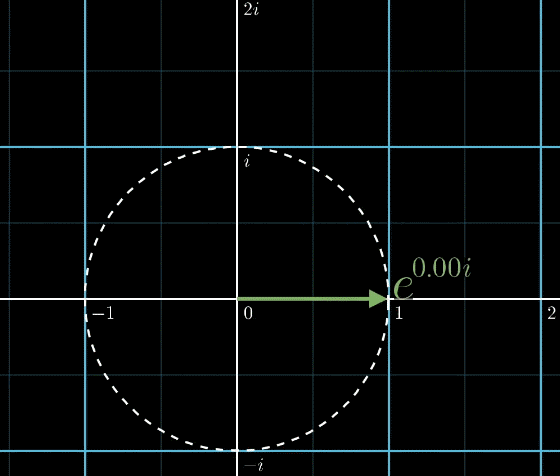#### 第二步：缠绕的表示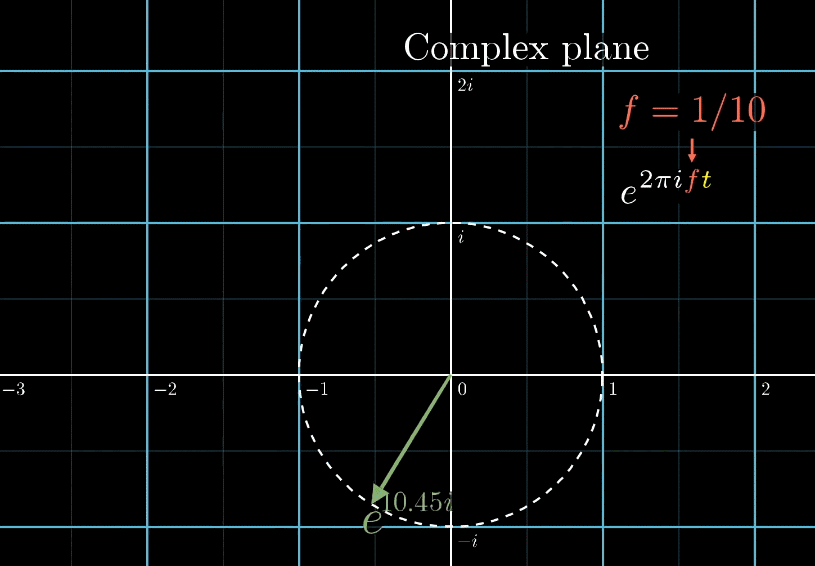#### 第三步：质心的表示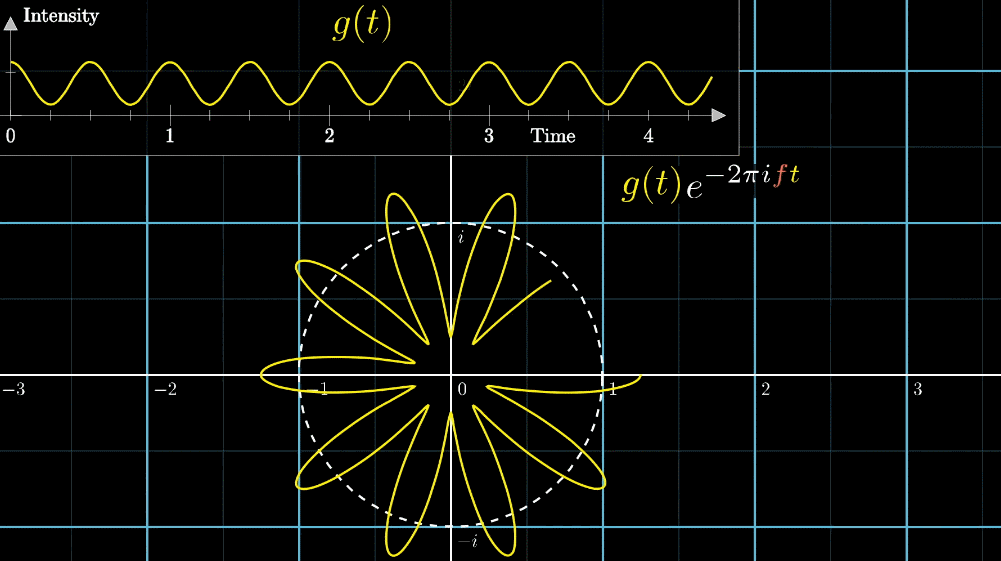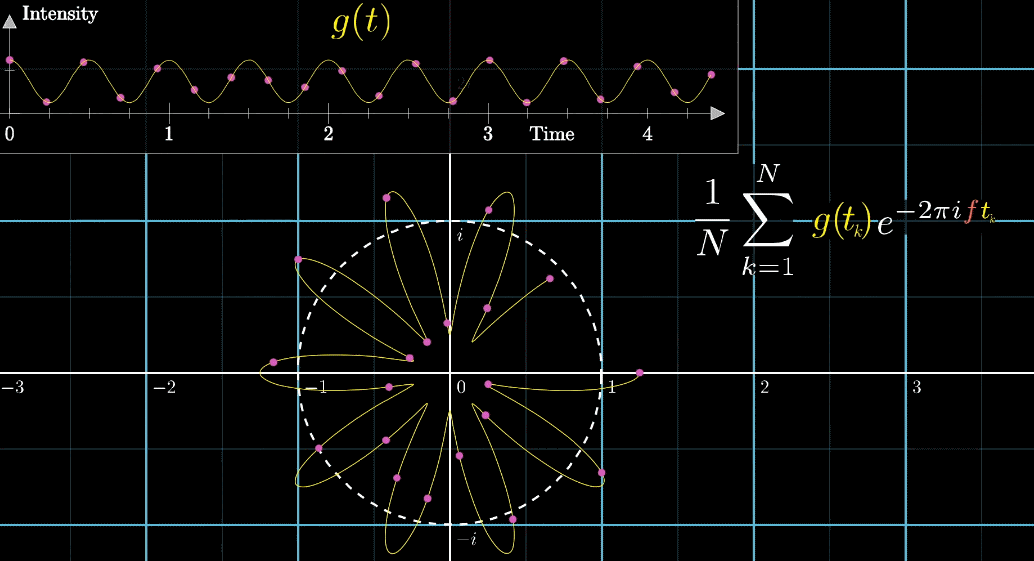#### 最终步：整理积分限和系数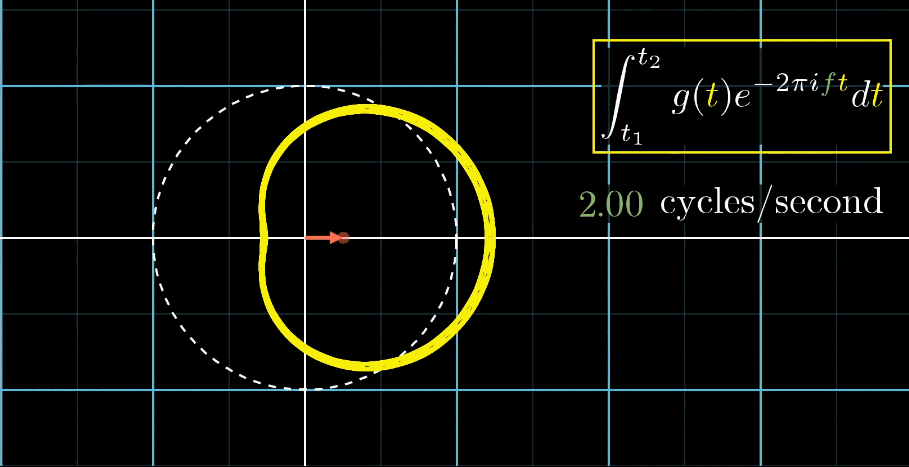## 补充

### 相位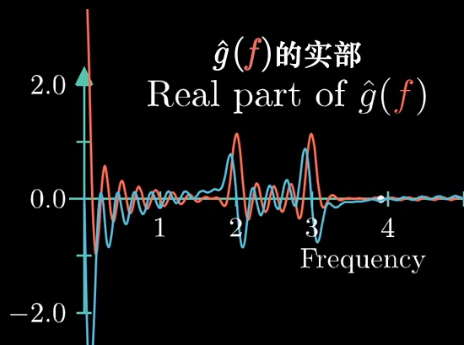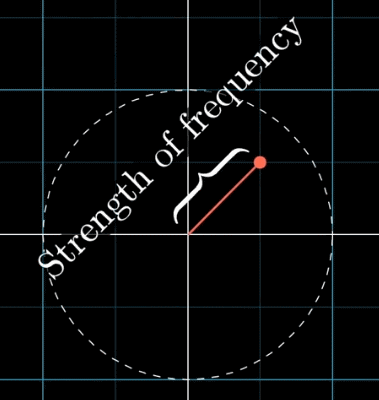### 原信号的长度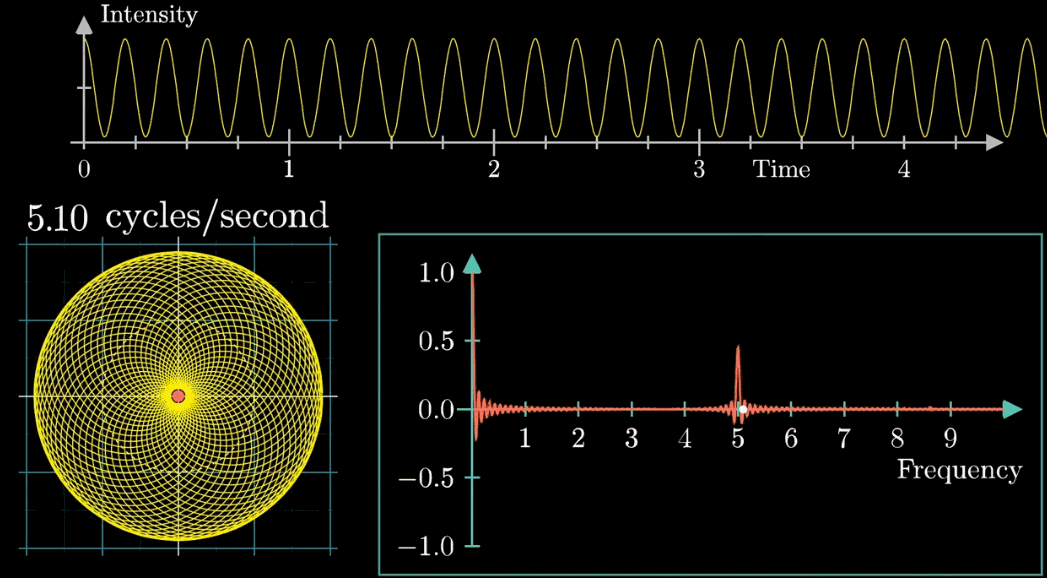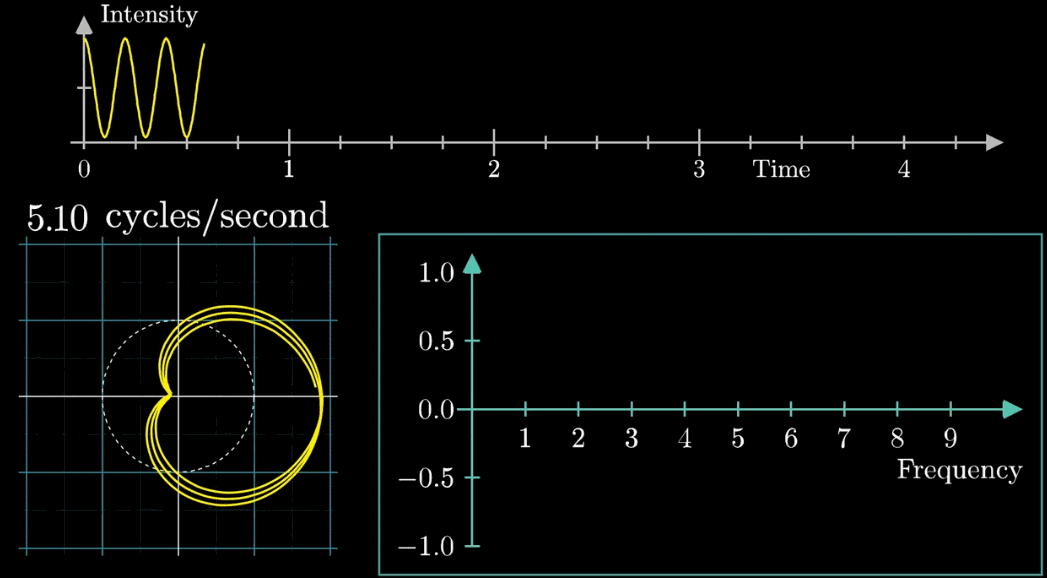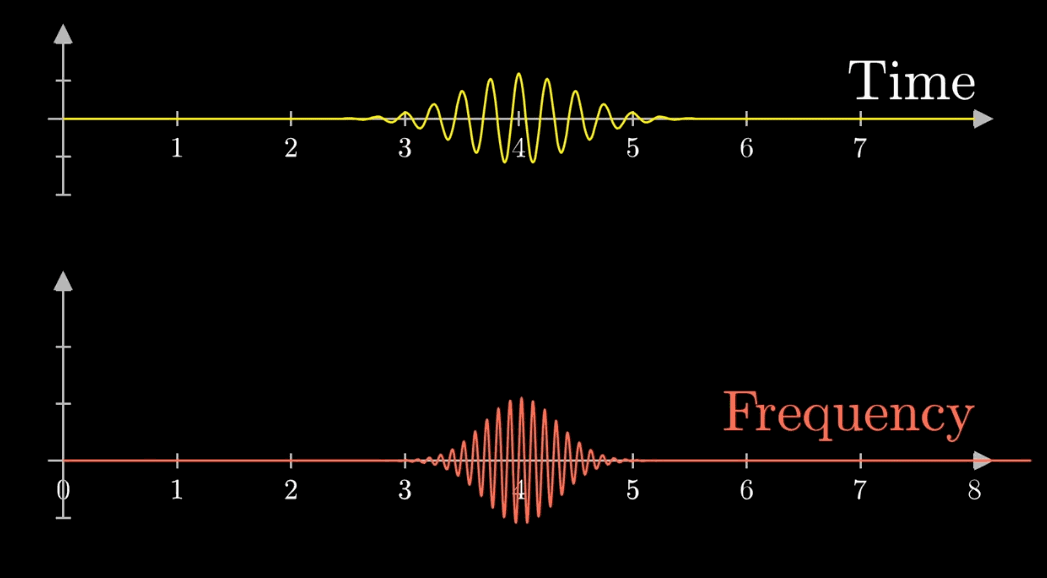# 总结• $e^{2\pi i}$ 表示单位圆，添加自变量即可表示旋转

• 与原函数相乘缠绕到单位圆

• 为求质心的特征，进行积分计算

• 一个逆时针旋转360°画成的圆 ➜ $e^{2\pi i}$

• 表示运动，需要原函数的自变量，时间 $t$ ➜ $e^{2 \pi i t}$

• 表示旋转速度，需要自变量，频率 $f$ ➜ $e^{2 \pi i f t}$

• 规定变换的采样方向为顺时针，加负号 ➜ $e^{-2 \pi ift}$

• 乘以原函数缠绕到单位圆并记录 ➜ $g(t) e^{-2 \pi ift}$ （此处使用 $g$ 符号标识原函数是为了和频率符号 $f$ 做区分）

• 为了计算质心特征，积分 ➜ $\int_{-\infty}^{+\infty} g(t)e^{-2\pi it \color{red}{f}}dt$

• 自变量为频率 $f$，写出函数表达式 ➜ $\hat g(\color{red}{f} \color{black}{) = \int_{-\infty}^{+\infty} g(t)e^{-2\pi it \color{red}{f}}dt}$